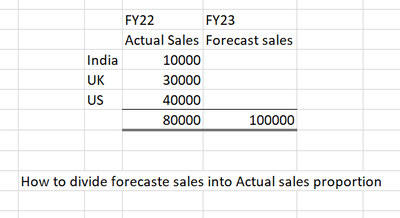# How to Calculate Forecast period revenue in proportion of actual sales of CYTagged:

•Hi, I belive you will need an intermediary line item with percentage calculation that you will use to multiply the forecast value by. So:

1. Create a Proportion Ratio by dividing country to the Total: Actual Sales/ Actual Sales Total (depending how you setup the list)

2. Refer the total forecast value in formula to multiply by the ratio.